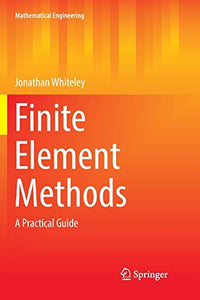# Finite Element Methods

Jonathan Whiteley

Format: Print Book

ISBN: 9783319842882

• SGD 218.39
Unit price per
• Save SGD 24.27
Tax included.

Will not ship until

This book presents practical applications of the finite element method to general differential equations. The underlying strategy of deriving the finite element solution is introduced using linear ordinary differential equations, thus allowing the basic concepts of the finite element solution to be introduced without being obscured by the additional mathematical detail required when applying this technique to partial differential equations. The author generalizes the presented approach to partial differential equations which include nonlinearities. The book also includes variations of the finite element method such as different classes of meshes and basic functions. Practical application of the theory is emphasised, with development of all concepts leading ultimately to a description of their computational implementation illustrated using Matlab functions. The target audience primarily comprises applied researchers and practitioners in engineering, but the book may also be beneficial for graduate students.

Publication Year: 2017
Imprint: Springer International Publishing
Format: P
Weight (Gram): 379